# Magnetic Dipole

## Magnetics of Class 12

A small current carrying loop acts like a magnetic dipole. The magnitude of a magnetic dipole moment or magnetic moment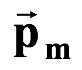. It is defined as the product of the current in a flat current - carrying loop and the area enclosed by it.

Thus,= I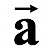The direction of magnetic moment coincides with the direction of the area vector (which is the direction of the magnetic field).

If the loop contains N number of turns, the magnetic moment is given by= NI(3.19)### Example: 3.12

A circular loop of wire of radius R carrying a current I is bent about its diameter along two mutually perpendicular planes as shown in the figure (3.20).

Find the magnetic moment of the loop.

##### Solution

The magnetic moment of the semicircle in the yz-plane is along the x-axis and that in the xz-plane is along the y-axis.

Thus,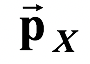=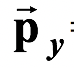=The total magnetic moment is given by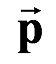= pxi+ py j

#### or=A.m2### Example : 3.13

 A circular ring of radius R carries a charge q uniformly distributed on its circumference. It is rotated at constant angular speed ω about the centroidal axis perpendicular to its plane. Find its magnetic moment.Solution

The motion of charges along with the ring produces current I which is given by

I =Thus, pm = IA =(πR2) = 1/2 ωqR2

Example : 3.14

A rotating rod is pivoted about one end and carries a total charge q uniformly distributed along its length L (fig. 3. 22 a). If the rod rotates with angular velocity ω, compute its magnetic moment μ.Solution

We visualize the rotating rod of charge as a series of concentric current loop, each carrying a current dI. The charge per unit length λ along the rod is

λ = q/L

Now dq = λdl and dI =λdl

The magnetic moment of this differential current loop is given by

dpm = dI (πl2) =πl2 =l2 dl

On integrating ,

Pm =Substituting for λ, we have

Pm =1 Electric Dipole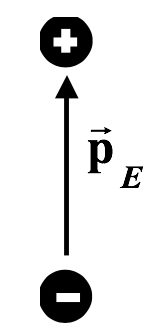Magnetic Dipole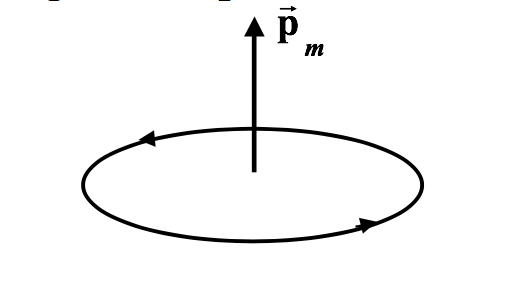2 Electric Field along the axis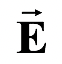|| =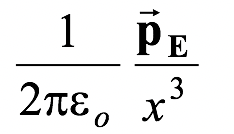Magnetic Field along the axis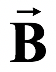|| =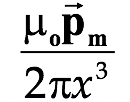3 Torque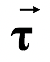=E ×Torque=m ×(3.20) 4 Potential Energy U = −E .Potential Energy U = −m.(3.21)

 Example: 3.15 The arrangement is same as shown in Fig. (3.23a ). (a) Find the potential energy of the loop (b) Find the work done, to increase the spacing between the wire and the loop from a to 2a. Solution (a) Since magnetic field is non-uniform over the loop therefore we cannot straight away use equation (3.21) to find the potential energy of the loop. A small loop of length l and width dx is assumed as shown in fig, (3.23 b). The magnetic moment of this loop is given by dpm = I2ldxThe direction of the magnetic moment is perpendicular to the plane of paper pointing inwards. Thus, the potential energy of this loop is given by

dU =-dm.= −dpmB

where B is the magnetic field at the position of this element

i.e. B =Thus, dU =The total potential energy is U =U =(b) The initial and final potential energies of the loop are

Ui =Ur =The work done is given by

W = Ur − Ui =